Courses

# Study of DC-AC Measuring Instruments (Part - 1) Electrical Engineering (EE) Notes | EduRev

## Electrical Engineering (EE) : Study of DC-AC Measuring Instruments (Part - 1) Electrical Engineering (EE) Notes | EduRev

The document Study of DC-AC Measuring Instruments (Part - 1) Electrical Engineering (EE) Notes | EduRev is a part of the Electrical Engineering (EE) Course Basic Electrical Technology.
All you need of Electrical Engineering (EE) at this link: Electrical Engineering (EE)

Objectives

• To understand the basic construction of a permanent magnet moving coil (PMMC) instrument and its operation. • Sketch and explain circuit diagrams for electromechanical dc ammeters and voltmeters.
• To understand how a single instrument may be used for multi-range ammeters and voltmeters.
• To study the advantage and limitation of PMMC instruments.
• To understand the basic construction of Moving-iron Instrument and its principle operation.
•  To study the advantage and errors involved in moving-iron instruments.

Introduction

Measuring instruments are classified according to both the quantity measured by the instrument and the principle of operation. Three general principles of operation are available: (i) electromagnetic, which utilizes the magnetic effects of electric currents; (ii) electrostatic, which utilizes the forces between electrically-charged conductors; (iii) electro-thermic, which utilizes the heating effect.

Electric measuring instruments and meters are used to indicate directly the value of current, voltage, power or energy. In this lesson, we will consider an electromechanical meter (input is as an electrical signal results mechanical force or torque as an output) that can be connected with additional suitable components in order to act as an ammeters and a voltmeter. The most common analogue instrument or meter is the permanent magnet moving coil instrument and it is used for measuring a dc current or voltage of a electric circuit. On the other hand, the indications of alternating current ammeters and voltmeters must represent the RMS values of the current, or voltage, respectively, applied to the instrument.

Various forces/torques required in measuring instruments

• Deflecting torque/force: The defection of any instrument is determined by the combined effect of the deflecting torque/force, control torque/force and damping torque/force. The value of deflecting torque must depend on the electrical signal to be measured; this torque/force causes the instrument movement to rotate from its zero position.
• Controlling torque/force: This torque/force must act in the opposite sense to the deflecting torque/force, and the movement will take up an equilibrium or definite position when the deflecting and controlling torque are equal in magnitude. Spiral springs or gravity usually provides the controlling torque.
• Damping torque/force: A damping force is required to act in a direction opposite to the movement of the moving system. This brings the moving system to rest at the deflected position reasonably quickly without any oscillation or very small oscillation.  This is provided by i) air friction ii) fluid friction iii) eddy current. It should be pointed out that any damping force shall not influence the steady state deflection produced by a given deflecting force or torque. Damping force increases with the angular velocity of the moving system, so that its effect is greatest when the rotation is rapid and zero when the system rotation is zero. Details of mathematical expressions for the above torques are considered in the description of various types of instruments.

General Theory Permanent Magnet Moving Coil (PMMC) Instruments

The general theory of moving-coil instruments may be dealt with considering a rectangular coil of N turns, free to rotate about a vertical axis.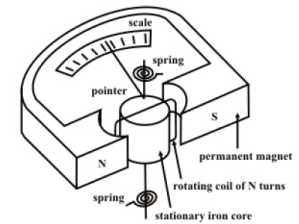FiK, 42.1(a): Permanent Magnet Moving Coll Instrument.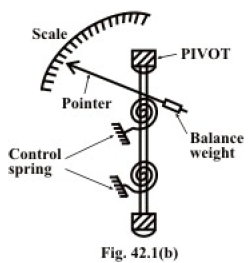Fig. 42.1(a) shows the basic construction of a PMMC instrument. A moving coil instrument consists basically of a permanent magnet to provide a magnetic field and a small lightweight coil is wound on a rectangular soft iron core that is free to rotate around its vertical axis. When a current is passed through the coil windings, a torque is developed on the coil by the interaction of the magnetic field and the field set up by the current in the coil. The aluminum pointer attached to rotating coil and the pointer moves around the calibrated scale indicates the deflection of the coil. To reduce parallax error a mirror is usually placed along with the scale. A balance weight is also attached to the pointer to counteract its weight (see Fig. 42.1(b)). To use PMMC device as a meter, two problems must be solved. First, a way must be found to return the coil to its original position when there is no current through the coil. Second, a method is needed to indicate the amount of coil movement.

The first problem is solved by the use of hairsprings attached to each end of the coil as shown in Fig. 42.1(a). These hairsprings are not only supplying a restoring torque but also provide an electric connection to the rotating coil. With the use of hairsprings, the coil will return to its initial position when no current is flowing though the coil. The springs will also resist the movement of coil when there is current through coil. When the developing force between the magnetic fields (from permanent magnet and electro magnet) is exactly equal to the force of the springs, the coil rotation will stop. The coil set up is supported on jeweled bearings in order to achieve free movement. Two other features are considered to increase the accuracy and efficiency of this meter movement. First, an iron core is placed inside the coil to concentrate the magnetic fields. Second, the curved pole faces ensure the turning force on the coil increases as the current increases.

It is assumed that the coil sides are situated in a uniform radial magnetic field of flux density B wb / m2 , let the length of a coil side (within the magnetic field) be l (meter), and the distance from each coil side to the axis be r (meter).

Principle of operation

It has been mentioned that the interaction between the induced field and the field produced by the permanent magnet causes a deflecting torque, which results in rotation of the coil. The deflecting torque produced is described below in mathematical form:

Deflecting Torque: If the coil is carrying a current of iamp. , the force on a coil side  = B il N (newton, N).                                                                     (42.1)

∴ Torque due to both coil sides = ( 2 r )(B i l N ) ( Nm)

= Gi ( Nm)                                                 (42.2)

where G is the Galvanometer constant and it is expressed as G = 2rBlN ( Nm / amp. )

= NBA ( Nm / amp. ). (note A = 2rl = area of the coil.)

N = no. of turns of the coil.

B = flux density in Wb / m2 Wb/m2.

l = length of the vertical side of the coil, m.

2 r = breadth of the coil, m

i = current in ampere.

A = 2rl = area, m2

Truly speaking, the equation (42.2) is valid while the iron core is cylindrical and the air gap between the coil and pole faces of the permanent magnet is uniform. This result the flux density B is constant and the torque is proportional to the coil current and instrument scale is linear.

Controlling Torque: The value of control torque depends on the mechanical design of the control device. For spiral springs and strip suspensions, the controlling torque is directly proportional to the angle of deflection of the coil.

i.e., Control torque = C θ                                                     (42.3)

where, θ = deflection angle in radians and C = spring constant Nm / rad.

Damping Torque: It is provided by the induced currents in a metal former or core on which the coil is wound or in the circuit of the coil itself. As the coil moves in the field of the permanent magnet, eddy currents are set up in the metal former or core. The magnetic field produced by the eddy currents opposes the motion of the coil. The pointer will therefore swing more slowly to its proper position and come to rest quickly with very little oscillation. Electromagnetic damping is caused by the induced effects in the moving coil as it rotates in magnetic field, provided the coil forms part of closed electric circuit.

Let the velocity of the coil is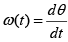red/ sec . d trad dt θ ω = , and let the resistance of the

coil circuit with N turns be R Ω .  Then the velocity of a coil side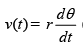(m/ sec .)

∴ E.m.f induced in each turn of the coil =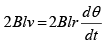volt = (note both the sides of the coil  having same e.m.fs but they are additive in nature).

∴ Induced current across N turns of coil =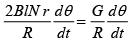amps.(R = resistance of coil)

By Lenz’s Law, torque produced =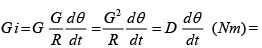opposing

torque. Note,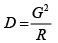is the damping constant for the induced currents in the coil due to its motion. This damping torque is active when the coil posses a change in deflection.  A metal former or core may be considered as a single-turn coil and if its dimensions are land r and its resistance R1.Similarly, damping torque for the former or core can be computed as

Damping torque (for the core or former) =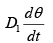(Nm) where D1damping constant due to induced currents in the core or former. In addition to the induced current damping, there will be a small damping torque due to air friction. Air damping torque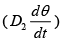may be assumed to be proportional to the angular velocity of the coil.

Equation of motion: The resulting torque in a coil or motion of a coil in a magnetic field is due to the combined effect of deflecting torque (Td) , controlling torque (CØ) damping torque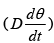and it is expressed mathematically as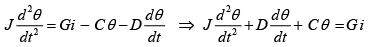where J is the moment of inertia of the moving parts. One can easily study the dynamic behavior of the above second order system by solving the differential equation.

Remarks: When the moving system reached at steady state i.e. at final deflected position, the controlling torque becomes equal and opposite to the deflecting torque. The deflecting angle is directly proportional to the current in the movable coil (see eq. 42.2). For this reason, the scale of the moving coil instrument is calibrated linearly.

A multi-range ammeters and voltmeters

An ammeter is required to measure the current in a circuit and it therefore connected in series with the components carrying the current. If the ammeter resistance is not very much smaller than the load resistance, the load current can be substantially altered by the inclusion of the ammeter in the circuit. To operate a moving coil instrument around a current level 50ma is impractical owing to the bulk and weight of the coil that would be required. So, it is necessary to extend the meter-range shunts (in case of ammeters) and multipliers (in case of volt meters) are used in the following manner.

For higher range ammeters a low resistance made up of manganin (low temperature coefficient of resistance) is connected in parallel to the moving coil (see Fig.42.2 (a)) and instrument may be calibrated to read directly to the total current.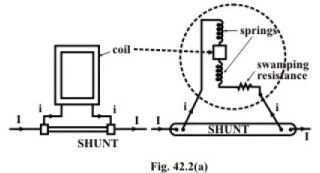They are called shunts. The movement of PMMC instrument is not inherently insensitive to temperature, but it may be temperature-compensated by the appropriate use of series and shunt resistors of copper and manganin. Both the magnetic field strength and springtension decrease with an increase in temperature. On other side, the coil resistance increases with an increase in temperature. These changes lead to make the pointer read low for a given current with respect to magnetic field strength and coil resistance. Use of manganin resistance (known as swamping resistance which has a temperature coefficient practically zero) in series with the coil resistance can reduce the error due to the variation of resistance of the moving coil. The swamping resistance (r ) is usually three times that of coil thereby reducing a possible error of, say, 4% to 1%. A multirange ammeter can be constructed simple by employing several values of shunt resistances, with a rotary switch to select the desired range. Fig. 42.2(b) shows the circuit arrangement.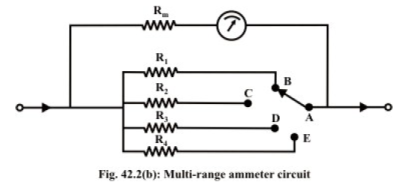When an instrument is used in this fashion, care must be taken to ensure shunt does not become open-circuited, even for a very short instant. When the switch is moved from position ‘ B ’ to ‘ C ’ or moved to any positions, the shunt resistance will remain opencircuited for a fraction of time, resulting a very large current may flow through the ammeter and damage the instrument. To avoid such situation, one may use the makebefore-break switch as shown in Fig.42.(c).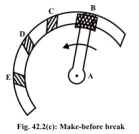The wide-ended moving contact connected to the next terminal to which it is being moved before it loses contact with the previous terminals. Thus, during the switching time there are two resistances are parallel with the instrument and finally the required shunt only will come in parallel to the instrument.

Example- L42.1 A PMMC instrument has a coil resistance of 100Ω and gives a fullscale deflection (FSD) for a current of 500 μ A . Determine the value of shunt resistance required if the instrument is to be employed as an ammeter with a FSD of 5A.

Solution: Let current flowing through the shunt is Is . From the circuit shown in Fig.42.3, one can the following expression using the parallel divided rule.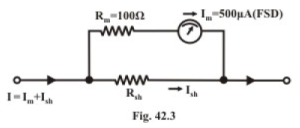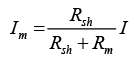whereRsh= shunt resis ce ,   Rm = ammeter resis tan ce =100Ω , Im = ammeter full scale deflection current = 500 μ A and I = desired range of ammeter = 5 A . From the above expression (42.4) we get,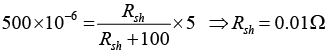Multi-range voltmeter: A dc voltmeter is constructed by a connecting a resistor in series with a PMMC instrument. Unlike an ammeter, a voltmeter should have a very high resistance Rse and it is normally connected in parallel with the circuit where the voltage is to be measured (see Fig.42.4). To minimize voltmeter loading, the voltmeter operating current should be very small i.e., the resistance connected in series with the coil should be high.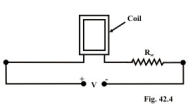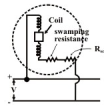The moving coil instruments can be suitably modified to act either as an ammeter or as a voltmeter.  For multi-range voltmeters the arrangement is as follows:  In Fig.42.5 any one of several multiplier resistors is selected by means of a rotary switch.  Unlike the case of the ammeter, the rotary switch used with the voltmeter should be a break-before make type, that is, moving contact should disconnect from one terminal before connecting to the next terminal.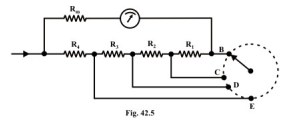Example-42.2 A PMMC meter with a coil resistance 100Ω and a full scale deflection current of 100 μ A is to be used in the voltmeter circuit as shown in Fig.42.6. The voltmeter ranges are to be 50 . Determine the required value of resistances for each range.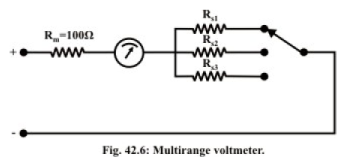Solution: From the circuit shown in Fig.42.6, one can write the expression  for the 50 V range as :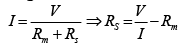Now for (i)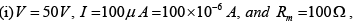the value of series

resistance (Rs2) = 0.4999M Ω.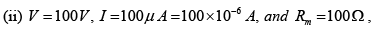the required value of resistance(Rs2) = 0.9999 M Ω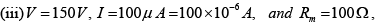the required series resistance (Rs2) =1.4999 M Ω .

Offer running on EduRev: Apply code STAYHOME200 to get INR 200 off on our premium plan EduRev Infinity!

57 docs|59 tests

,

,

,

,

,

,

,

,

,

,

,

,

,

,

,

,

,

,

,

,

,

;